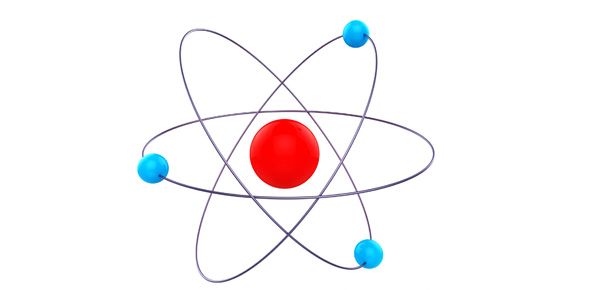# Chem Mastery Formula Mass Set 1

3 QuestionsSettingsRelated Topics
• 1.
What is the approximate molecular/formula mass of the following compound: Al(NO3)3
• A.

210

• B.

211

• C.

212

• D.

213

• E.

214

• F.

215

• G.

216

• 2.
What is the approximate molecular/formula mass of the following compound: Fe(HCO3)3
• A.

237

• B.

239

• C.

241

• D.

243

• E.

245

• 3.
What is the approximate molecular/formula mass of the following compound: CuSO4.5H2O
• A.

90

• B.

160

• C.

180

• D.

230

• E.

250

• F.

270